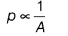# Differentiate between thrust and pressure. How does 1 Pascal differ from 1 Newton?

1. Differentiate between thrust and pressure.
2. How does 1 Pascal differ from 1 Newton?
1. Difference between thrust and pressure are
Thrust Pressure
The force acting on a body when it is partially or fully immersed in a fluid. The force per unit area, acting perpendicular to the surface.
Its SI unit is Newton. Its SI unit is N/m2 or Pa (Pascal).
1. The pressure exerted by 1 N of force which is acting perpendicular to the surface, if area 1 m2 is called Pascal.
∴ 1Pa = 1N/m2
Force required to accelerate 1 kg mass at the rate of 1 m/s2 is called Newton.
1N = 1 kg-m/s2
Since, we know that
Pressure, p = F/AIf area is doubled, then pressure will reduce to half of its initial value.